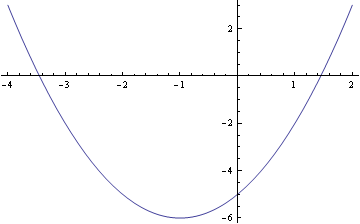Where Is The Vertex Of The Parabola Y = X2 + 2x - 5?

What is the vertex of x^2+x-5=0
thanked the writer.
The vertex of parabola ax2+bx+c is located at x=-b/(2a).

Your parabola has a=1, b=2, so the vertex is located at x=-2/2 = -1

y = (-1)2 + 2(-1) -5 = 1 -2 -5 = -6

The coordinates of the vertex are (-1, -6)

(Look at the picture.)thanked the writer.
Y = 6(x+2)^2 - 4
thanked the writer.
Y = x^2+2x-5....(1)
The vertex of parabola for ax^2+bx+c is x = -b/2a
so, a=1, b=2, c=-5
x = -2/(2*1)
x = -2/2 = -1
put the value of x into eq. (1)
y = (-1)^2+2(-1)-5
y = 1-2-5
y = -6
so, points for vertex of parabola are : (-1, -6)
thanked the writer.